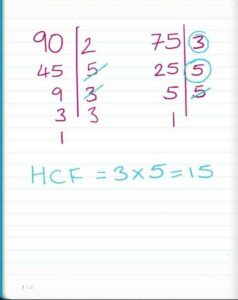# HCF Of Two & N Numbers Java Program | 3 Ways

Java program to find HCF of two numbers – The below given Java programs explains the process of evaluating the Highest Common Factor(HCF) between two given numbers.

The methods used to find the HCF of two numbers is Java Programming is as follows:

• Using Command Line Arguments
• Using Static method
• Using Recursion

In the end, we will discuss how to find the HCF of n numbers. We do also add compiler for each and every program at the end of the article, with proper examples and sample outputs.

What Is HCF?

HCF or Highest Common Factor is the greatest common divisor between two or more given numbers.

For example:

Let there be two arbitrary numbers such as 75 and 90.

75 = 3 * 5 * 5

90 = 2 * 3 * 3 * 5

Common Divisor = 3 * 5 = 15

Here, the HCF of the three given numbers would be 15 since it divides every given number without leaving a fraction behind.## HCF Of Two Numbers – Command Line Arguments

1) The values we will pass at run-time are called command line arguments.

2) n1=Integer.parseInt(arg); here reading the first value from string array which is at index “0”, and converting the string value into an integer using parseInt() method.

n2=Integer.parseInt(arg); here reading the 2nd value from string which is at index “1” and converting string value into integer using parseInt() method. Here Integer is “wrapper” class.

3) The while loop iterates until n2>0 is false temp assigned to n2, n2 assigned to the remainder of n1/n2,  n1 assigned to n1. If the condition fails then it prints the HCF of two numbers is n1.

4) In our example, we are passing 15, 35 as command line arguments, and converted as integers and store the values at n1=15, n2=35.    while loop checks the condition  35>0 is true , temp=35, n2=5, n1=35, 5>o true,temp=5,n2=0,n1=5. For n2=0 condition fails and prints the HCF is 5.

Output:

### Using Static Method

1. Calls the static hcfCal() method in the main method, the method executes the code and prints the value.

Output:

#### Using Recursion

1) In this program, the method highest(int a, int b) calls itself as highest(a,b).

2) Using scanner object, read the values and store in the variables n1, n2. Using Hcf class object call the method highest(n1,n2), the method starts the execution and calls itself, it repeats until the condition if(a!=b) is false.

Output:

##### Finding HCF Of n numbers

1) We are finding the HCF of n numbers by passing each element of an array to the function hcf(result, input[i]) using for loop with the structure for(int i = 1; i < input.length; i++).

Output:

More Programs:

x

## Plus Star Pattern Java Program | Patterns

Java program to print plus star pattern program – We have written the below print/draw ...